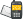It is currently 26 May 2019, 21:02### GMAT Club Daily Prep

#### Thank you for using the timer - this advanced tool can estimate your performance and suggest more practice questions. We have subscribed you to Daily Prep Questions via email.

Customized
for You

we will pick new questions that match your level based on your Timer History

Track
Your Progress

every week, we’ll send you an estimated GMAT score based on your performance

Practice
Pays

we will pick new questions that match your level based on your Timer History

#### Not interested in getting valuable practice questions and articles delivered to your email? No problem, unsubscribe here.# Given x,y,z are distinct positive real numbersQuestion banks Downloads My Bookmarks Reviews Important topics
Author Message
TAGS:
ManagerJoined: 12 Jan 2016
Posts: 143
Followers: 1

Kudos [?]: 73 , given: 17

Given x,y,z are distinct positive real numbers [#permalink]00:00

Question Stats:53% (01:01) correct46% (02:07) wrongbased on 13 sessions
Given x,y,z are distinct positive real numbers

 Quantity A Quantity B (x²(y+z)+y²(x+z)+z²(x+y))/(xyz) 6

A)The quantity in Column A is greater.
B)The quantity in Column B is greater.
C)The two quantities are equal.
D)The relationship cannot be determined from the information given.
[Reveal] Spoiler: OA

Last edited by Sonalika42 on 25 Sep 2016, 03:23, edited 2 times in total.
InternJoined: 02 Jul 2016
Posts: 25
Followers: 0

Kudos [?]: 15 , given: 2

Re: Given x,y,z are distinct positive real numbers [#permalink]
D is the answer

Posted from my mobile deviceGRE Prep Club LegendJoined: 07 Jun 2014
Posts: 4857
GRE 1: Q167 V156WE: Business Development (Energy and Utilities)
Followers: 112

Kudos [?]: 1865 , given: 397

Re: Given x,y,z are distinct positive real numbers [#permalink]
Expert's post
The best way to approach this problem is by substitution.

Quantity A is $$\frac{(x^2(y+z)+y^2(x+z)+z^2(x+y))}{(xyz)}$$

or $$x(\frac{1}{y}+\frac{1}{z})+y(\frac{1}{x}+\frac{1}{z})+z(\frac{1}{x}+\frac{1}{y})$$

or $$\frac{x}{y}+\frac{x}{z}+\frac{y}{x}+\frac{y}{z}+\frac{z}{y}+\frac{z}{x}$$

rearrange

$$\frac{x}{y}+\frac{y}{x}+\frac{x}{z}+\frac{z}{x}+\frac{z}{y}+\frac{y}{z}$$.

now Let $$\frac{x}{y}$$ = n. Then the first two terms become $$n + \frac{1}{n}$$. The minimum value of $$n + \frac{1}{n}$$ is 2 when n =1. Or and n can be one if x=y.

Hence a case x=y=z= 5( or any other real positive number) is not possible. Hence Quantity A is always greater

for x=1 y=1 z=2

$$\frac{1}{1}+\frac{1}{1}+\frac{1}{2}+\frac{2}{1}+\frac{2}{1}+\frac{1}{2}$$

$$1+1+0.5+2+2+0.5$$ =$$7$$. Now Quantity A is 7.

Hence A is the correct option.
_________________

Sandy
If you found this post useful, please let me know by pressing the Kudos Button

Try our free Online GRE TestInternJoined: 21 Jun 2016
Posts: 1
Followers: 0

Kudos [?]: 1  , given: 0

Re: Given x,y,z are distinct positive real numbers [#permalink]
1
This post received
KUDOS
x,y and z are said to be distinct positive real number.So you can not make x,y or z values equal i think,sandy.I guess the answer to be A.
GRE Prep Club LegendJoined: 07 Jun 2014
Posts: 4857
GRE 1: Q167 V156WE: Business Development (Energy and Utilities)
Followers: 112

Kudos [?]: 1865 , given: 397

Re: Given x,y,z are distinct positive real numbers [#permalink]
Expert's post
siam1993 wrote:
x,y and z are said to be distinct positive real number.So you can not make x,y or z values equal i think,sandy.I guess the answer to be A.

Hey,

Yeah you are correct. Missed the distinct part. Changed the answer.

Regards,
_________________

Sandy
If you found this post useful, please let me know by pressing the Kudos Button

Try our free Online GRE Test

ManagerJoined: 27 Sep 2017
Posts: 112
Followers: 1

Kudos [?]: 30 , given: 4

Re: Given x,y,z are distinct positive real numbers [#permalink]
Sonalika42 wrote:
Given x,y,z are distinct positive real numbers

 Quantity A Quantity B (x²(y+z)+y²(x+z)+z²(x+y))/(xyz) 6

A)The quantity in Column A is greater.
B)The quantity in Column B is greater.
C)The two quantities are equal.
D)The relationship cannot be determined from the information given.

My problem would be more like, since x, y, z are positive numbers which does NOT specify integers. Then it would be that x=0.2 y= 1 z= 2, though it seems that ans should be A. but can we guarantee there is no excpetion?
InternJoined: 15 Mar 2018
Posts: 32
Followers: 0

Kudos [?]: 8 , given: 1

Re: Given x,y,z are distinct positive real numbers [#permalink]
Incorrect question, what if X Y Z are -1 0 1 respectively, the A will be undefined?

Zero is a real number .
ManagerJoined: 27 Sep 2017
Posts: 112
Followers: 1

Kudos [?]: 30 , given: 4

Re: Given x,y,z are distinct positive real numbers [#permalink]
HEcom wrote:
Incorrect question, what if X Y Z are -1 0 1 respectively, the A will be undefined?

Zero is a real number .

pay attention to distinct "positive" real numbers

0 is not - or +
InternJoined: 23 Nov 2017
Posts: 45
Followers: 0

Kudos [?]: 46 , given: 0

Re: Given x,y,z are distinct positive real numbers [#permalink]
HEcom wrote:
Incorrect question, what if X Y Z are -1 0 1 respectively, the A will be undefined?

Zero is a real number .

Just for clarification. Zero is a real number but it is neither positive nor negative. The very definition of a positive number is a number greater than zero and the definition of a negative number is a number less than zero.Re: Given x,y,z are distinct positive real numbers   [#permalink] 29 Mar 2018, 13:04
Display posts from previous: Sort by

# Given x,y,z are distinct positive real numbersQuestion banks Downloads My Bookmarks Reviews Important topicsPowered by phpBB © phpBB Group Kindly note that the GRE® test is a registered trademark of the Educational Testing Service®, and this site has neither been reviewed nor endorsed by ETS®.